Next: The Relaxation Function Up: Measuring the penetration depth with TF-SR Previous: The Raw Asymmetry For 2-Counter Geometry

# The Corrected Asymmetry

In practice, differences in counter efficiency and deviations from perfect geometry complicate the above treatment. If the sensitivity of the individual positron counters are not the same, the values of the normalization constant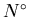, the maximum decay asymmetry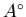and the random background levels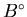will differ. The number of recorded events in each counter also depends on the solid angle they subtend at the sample. If the coverage of the solid angle is not maximized because of counter misalignments, there will be a decrease in the number of decay positrons detected. To account for these differences one can modify the above results by assuming: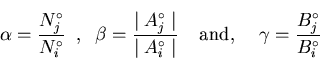(29)

Experimentally one can determine these constants by examining data above Tc where the muon precesses around a spatially constant field. With the above considerations, the raw asymmetry Aij(t) for the counters i and jcan then replaced by a corrected asymmetry Acorrected(t). This is obtained by substituting Eq. (3.29) into Eq. (3.20) and defining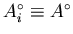and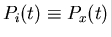: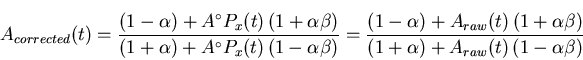(30)Next: The Relaxation Function Up: Measuring the penetration depth with TF-SR Previous: The Raw Asymmetry For 2-Counter Geometry
Jess H. Brewer
2001-09-28